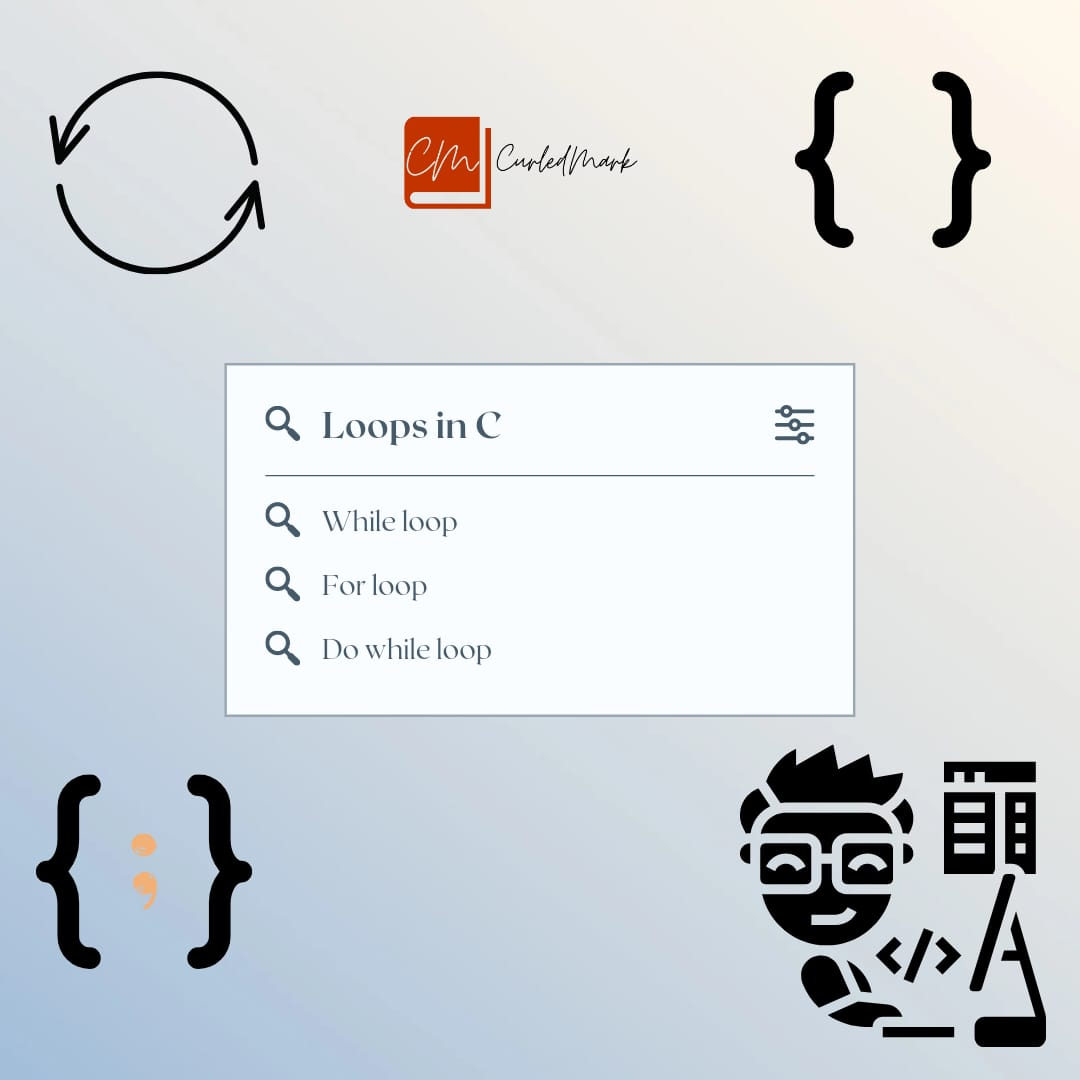September 25, 2022# Write programs of searching concepts || Search program

We will discuss the algorithms for searching programs. Three types of “searching” programs are given in this article. By understanding the logic and executing the program you will know the concepts of searching programming. You will get exposure to a search program in different styles.

## Accept 10 numbers and the number to search(lookFor)

The output would be Found or Not Found.

#include<stdio.h>

int main()

{

int x,y,lookFor,Found;

y=0;

While(y<=9)

{

Printf(“Enter a number:”);

Scanf(“%d”,&x[y]);

y++;

}

Printf(“Enter the number to search: “);

Scanf(“%d”,&lookFor);

found=0;

While(y<=9)

{

if(lookFor == x[y])

{

found=1;

break;

}

y++;

}

if(found == 0)

{

}

else

{

Printf(%d found”,lookFor);

}

return 0;

}

## Output

`Enter a number: 15`

`Enter a number: 8`

`Enter a number: 70`

`Enter a number: 43`

`Enter a number: 59`

`Enter a number: 44`

`Enter a number: 33`

`Enter a number: 25`

`Enter a number: 18`

`Enter the number to search: 2`

`Enter a number: 15`

`Enter a number: 8`

`Enter a number: 70`

`Enter a number: 43`

`Enter a number: 59`

`Enter a number: 44`

`Enter a number: 33`

`Enter a number: 25`

`Enter a number: 18`

`Enter the number to search: 70`

70 found

## Explanation of Search program

The first while loop is to accept 10 numbers from the keyboard. Also, we have accepted the number to search from the keyboard in the next line in the “printf” statement. We can then traverse the array to search for the number stored in “lookFor” variable. if it matches with one of the numbers in the array that is x[y] then found equals 1. This means we are declaring 1 when it is true and 0 when false similar to the Prime number program. If found is 0 then the number is not found else found.

Prime, reverse,power Programs in C Programming

## Accept 10 numbers and the number to search(lookFor)

The output would be Not Found or Found at index 7.

#include<stdio.h>

int main()

{

int x,y,lookFor,Found;

y=0;

While(y<=9)

{

Printf(“Enter a number:”);

Scanf(“%d”,&x[y]);

y++;

}

Printf(“Enter the number to search: “);

Scanf(“%d”,&lookFor);

found=0;

While(y<=9)

{

if(lookFor == x[y])

{

found=1;

break;

}

y++;

}

if(found == 0)

{

}

else

{

Printf(%d found at index %d”,lookFor,y);

}

return 0;

}

## Output

`Enter a number: 15`

`Enter a number: 8`

`Enter a number: 70`

`Enter a number: 43`

`Enter a number: 59`

`Enter a number: 44`

`Enter a number: 33`

`Enter a number: 25`

`Enter a number: 18`

`Enter a number: 1`

`Enter the number to search: 2`

`Enter a number: 15`

`Enter a number: 8`

`Enter a number: 70`

`Enter a number: 43`

`Enter a number: 59`

`Enter a number: 44`

`Enter a number: 33`

`Enter a number: 25`

`Enter a number: 18`

`Enter a number: 1`

`Enter the number to search: 25`

25 found at index 7

## Explanation of Search Program

The first while loop is to accept 10 numbers from the keyboard. Also, we have accepted the number to search from the keyboard in the next line in the “printf” statement. We can then traverse the array to search for the number stored in “lookFor” variable. if it matches with one of the numbers in the array that is x[y] then found equals 1. This means we are declaring 1 when it is true and 0 when false similar to the Prime number program. If found is 0 then the number is not found else found.

Everything is the same as the previous program except for the printing. The requirement is that the output would be Not Found or Found at index 7.

## Accept 10 numbers and the number to search(lookFor)

The output: Count how many times the searched number is found

Algorithm:

count=0;

y=0;

While(y<=9)

{

if(lookFor==x[y])

{

count++;

}

y++;

}

### Program on Search Program

#include<stdio.h>

int main()

{

int x,y,lookFor,count;

y=0;

While(y<=9)

{

Printf(“Enter a number:”);

Scanf(“%d”,&x[y]);

y++;

}

Printf(“Enter the number to search and count: “);

Scanf(“%d”,&lookFor);

count=0;

y=0;

While(y<=9)

{

if(lookFor==x[y])

{

count++;

}

y++;

}

Printf(“Searched for %d\n”,lookFor);

Printf(“The count is %d\n”,count);

}

return 0;

}

`Enter a number: 15`

`Enter a number: 8`

`Enter a number: 70`

`Enter a number: 4`

`Enter a number: 59`

`Enter a number: 4`

`Enter a number: 33`

`Enter a number: 25`

`Enter a number: 18`

`Enter a number: 4`

`Enter the number to search: 4`

Searched for 4

The count is 3

## Explanation of Search Program

The first while loop is to accept 10 numbers from the keyboard. Also, we have accepted the number to search from the keyboard in the next line in the “printf” statement. We can then traverse the array to search for the number stored in “lookFor” variable.

The requirement is quite different in this program. When the number is found with the help of the count variable we are counting the number of times the searched number is found.

## Conclusion of Search Programs

All the programs are super simple. Try to understand, you will get it easily. Read the explanation for more clarification and stay tuned for the upcoming blogs.

https://curledmark.in/the-largest-number-program-in-an-array-in-c-programming/

https://curledmark.in/armstrong-number-and-arrays-in-c-programming/

https://curledmark.in/prime-reversepower-programs-in-c-programming/

The Book Of Five Rings Summary

The Blue Umbrella

How to Talk to Anyone by Leil Lowndes

Jonathan Livingston Seagull Book Summary

Your Brain on PORN Book Summary by Gary Wilson

THE IMMORTALS OF MELUHA By Amish Tripathi full summary

Eleven Minutes by Paulo Coelho Summary

Hyperfocus by Chris Bailey Summary

Black Beauty by Anna Sewell Summary

You Can Heal Your Life by Louise Hay Summary

ATTITUDE IS EVERYTHING BY JEFF KELLER SUMMARY

THE BIOGRAPHY OF SWAMI VIVEKANANDA

THE SHAWSHANK REDEMPTION EXPLAINED

Turtles All the Way Down by John Green Story Explained

#### You may have missed#### What is do-while loop in C Programming ? Loops